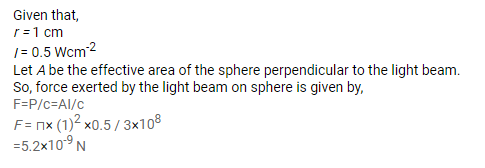# A sphere of radius 1.00 cm is placed in the path of a parallel beam of

Question:

A sphere of radius $1.00 \mathrm{~cm}$ is placed in the path of a parallel beam of light of large aperture. The intensity of the light is $0.5 \mathrm{~W}$ $\mathrm{cm}^{-2}$. If the sphere completely absorbs the radiation falling on it, find the force exerted by the light beam on the sphere.

Solution: